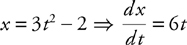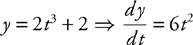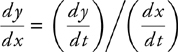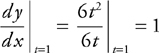# AP Calculus BC Question 330: Answer and Explanation

### Test Information

Question: 330

5. Which of the following is an equation of the line tangent to the curve with parametric equations x = 3t2 - 2, y = 2t3 + 2 at the point when t = 1?

• A. y = 3x2 + 7x
• B. y = 6x - 2
• C. y = x
• D. y = x + 3and, and sinceis the slope of the tangent line. At t = 1, x = 1, y = 4, so the point of tangency is (1, 4). Equation of tangent: y - 4 = 1(x - 1) ⇒ y = x + 3.